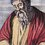# Can we define parity & primality for negative numbers?

1st Part: Can we define a negative number as even or odd?

I think it would be yes because any negative number when divided by 2 either leaves remainder of 0 and 1. I need suggestions about it.

2nd Part: The second part is tricky: Can we define a negative number to be prime or Composite?

If I define positive prime number as follows: An positive Integer having exactly two positive distinct factors. Then 2, 3, 5, 7,.... would be prime and 0, 1, 4, 6,.... would not be prime. If we take -2, it would have infinitely distinct factorizations: -2 = (-1)(2); -2 = (1)(-2); -2 = (-1)(-1)(-1)(2);...... and it doesn't obey fundamental theorem of Arithmetic since it isn't unique. Similarly taking prime factorizations of any negative Integer always give multiple factorizations. So, negative numbers couldn't be defined to be prime or composite in the same way as positive Integer greater than 1.

My real question is 'If I want to define "Negative Prime" in a different way, then how can I do it'?3 years, 2 months ago

This discussion board is a place to discuss our Daily Challenges and the math and science related to those challenges. Explanations are more than just a solution — they should explain the steps and thinking strategies that you used to obtain the solution. Comments should further the discussion of math and science.

When posting on Brilliant:

• Use the emojis to react to an explanation, whether you're congratulating a job well done , or just really confused .
• Ask specific questions about the challenge or the steps in somebody's explanation. Well-posed questions can add a lot to the discussion, but posting "I don't understand!" doesn't help anyone.
• Try to contribute something new to the discussion, whether it is an extension, generalization or other idea related to the challenge.

MarkdownAppears as
*italics* or _italics_ italics
**bold** or __bold__ bold
- bulleted- list
• bulleted
• list
1. numbered2. list
1. numbered
2. list
Note: you must add a full line of space before and after lists for them to show up correctly
paragraph 1paragraph 2

paragraph 1

paragraph 2

[example link](https://brilliant.org)example link
> This is a quote
This is a quote
    # I indented these lines
# 4 spaces, and now they show
# up as a code block.

print "hello world"
# I indented these lines
# 4 spaces, and now they show
# up as a code block.

print "hello world"
MathAppears as
Remember to wrap math in $$ ... $$ or $ ... $ to ensure proper formatting.
2 \times 3 $2 \times 3$
2^{34} $2^{34}$
a_{i-1} $a_{i-1}$
\frac{2}{3} $\frac{2}{3}$
\sqrt{2} $\sqrt{2}$
\sum_{i=1}^3 $\sum_{i=1}^3$
\sin \theta $\sin \theta$
\boxed{123} $\boxed{123}$

Sort by:

Yes, you can do all that. If you study ring theory, these things are well understood.

- 3 years, 2 months ago